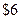Chapter 8, Problem 4SCQ### Principles of Economics 2e

2nd Edition
Steven A. Greenlaw; David Shapiro
ISBN: 9781947172364

#### Solutions

Chapter
Section### Principles of Economics 2e

2nd Edition
Steven A. Greenlaw; David Shapiro
ISBN: 9781947172364
Textbook Problem

# Suppose that the market price increases toas Table 8.14 shows. What would happen to the profit-maximizing output level?To determine

The effect on the profit maximizing level of output if the price rises to $6. Explanation If price is$6 for a perfectly competitive firm, as per table, the profit maximizing quantity will have a marginal cost of $6 also. By looking into the table, we can see that, 90 units of output have a marginal cost of$6.

Now, calculating profit at this output level:

Profit = Total Revenue - Tot

### Still sussing out bartleby?

Check out a sample textbook solution.

See a sample solution

#### The Solution to Your Study Problems

Bartleby provides explanations to thousands of textbook problems written by our experts, many with advanced degrees!

Get Started

#### Find more solutions based on key concepts